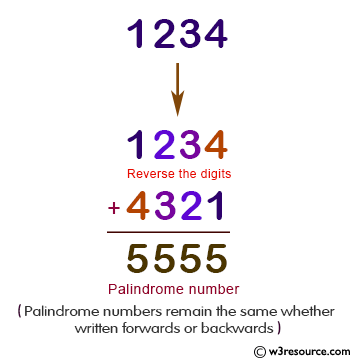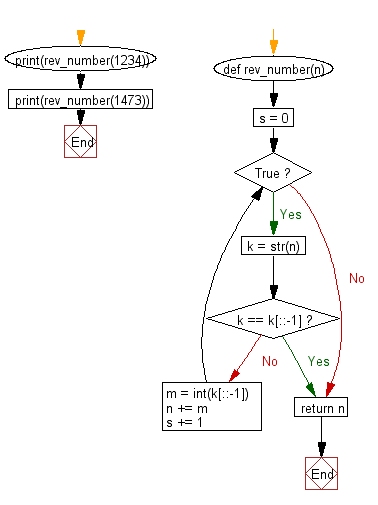﻿ Python: Reverse the digits of a given number and add it to the original - w3resource# Python: Reverse the digits of a given number and add it to the original

## Python Basic - 1: Exercise-30 with Solution

Write a Python program to reverse the digits of a given number and add it to the original, If the sum is not a palindrome repeat this procedure.

Note: A palindrome is a word, number, or other sequence of characters which reads the same backward as forward, such as madam or racecar.

Pictorial Presentation:Sample Solution:

Python Code:

``````def rev_number(n):
s = 0
while True:
k = str(n)
if k == k[::-1]:
break
else:
m = int(k[::-1])
n += m
s += 1
return n

print(rev_number(1234))
print(rev_number(1473))
``````

Sample Output:

```5555
9339
```

Flowchart:## Visualize Python code execution:

The following tool visualize what the computer is doing step-by-step as it executes the said program:

Python Code Editor:

Have another way to solve this solution? Contribute your code (and comments) through Disqus.

What is the difficulty level of this exercise?

Test your Programming skills with w3resource's quiz.

﻿

## Python: Tips of the Day

Iterating over dictionaries using 'for' loops:

I am a bit puzzled by the following code: d = {'x': 1, 'y': 2, 'z': 3} for key in d: print key, 'corresponds to', d[key] What I don't understand is the key portion. How does Python recognize ...

key is just a variable name.

```for key in d:
```

For Python 3.x:

```for key, value in d.items():
```

For Python 2.x:

```for key, value in d.iteritems():
```

To test for yourself, change the word key to poop.

In Python 3.x, iteritems() was replaced with simply items(), which returns a set-like view backed by the dict, like iteritems() but even better. This is also available in 2.7 as viewitems().

The operation items() will work for both 2 and 3, but in 2 it will return a list of the dictionary's (key, value) pairs, which will not reflect changes to the dict that happen after the items() call. If you want the 2.x behavior in 3.x, you can call list(d.items()).

Ref: https://bit.ly/37dm0Qo